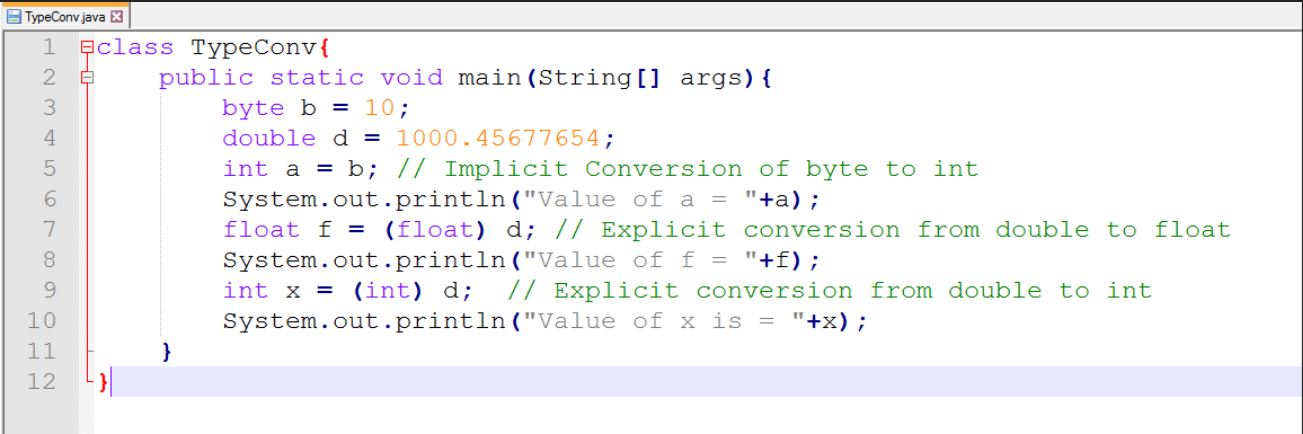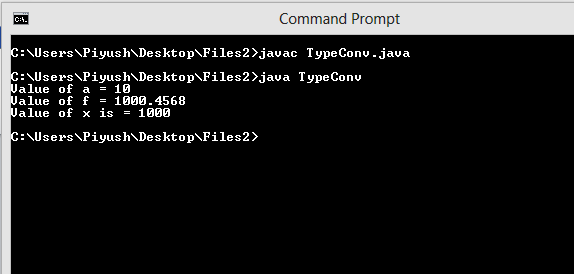# CORE JAVA TYPE CONVERSION

Type Conversion In Java | Core Java Tutorial | Minigranth

# Type Conversion In Java : Introduction

• Type conversion in java is the technique of converting one type (data-type) into other type.
• Type conversion in java can be done between compatible types and incompatible types. Compatible types are those which can be implicitly converted.
• For example : Conversion from byte to int is compatible, since int can hold a byte easily.
• Incompatible types are those which need to be explicitly converted by casting.
• For example : Conversion from int to byte is incompatible, since a byte is smaller than int and cannot hold it.
• Hence there are two types of type conversion in java :
• Implicit Type Conversion
• Explicit Type Conversion

### Type Conversion In Java : The Discussion

• With type conversion being such an important topic, Let us discuss the two type conversion in java in details along with a sample program below.
1. Implicit Type Conversion
• As discussed implicit type conversion occurs for compatible types. Implicit type conversion is done automatically, and the destination data type should be larger is size than the source data type. Hence a widening conversion takes place.
• For example : Type Conversion from a float to a double is implicit type conversion.
2. Explicit Type Conversion
• Explicit type conversion occurs for incompatible types.
• Explicit type conversion is done forcefully by casting a larger type to smaller type. Since we are converting a large type to a smaller type, this conversion is also called narrowing conversion.
• Syntax for explicit casting can be defined as,

 (required-type) value

• In the syntax required-type indicates the type to which the type of the value is to be converted. Value is the value of some variable.
• For example : Converting an int to a byte is done explicitly by casting int to byte.

 int a = 10; byte b = (byte) a ;  //Casting

### Type Conversion In Java : Example

• Let us take an example to discuss both implicit and explicit type conversion:Type Conversion In Java : ExampleType Conversion In Java : Output

• In the above output the value of double when casted to a float is truncated. This is because of the smaller size of float than double.
• Similarly conversion from double to int also truncates the fractional part as int only hold integer value.